# Battle of Heavyweights: VLOOKUPThis is the first post in a series called Battle of Heavyweights. In the series, we’ll use a boxing metaphor to compare VLOOKUP and SUMIFS. Now, as you read the next paragraph, use your best announcer’s voice and be sure to imagine the roar of a cheering crowd 🙂

“Ladies and gentlemen, welcome to the main event of the evening. Live from Las Vegas, we have a true battle of heavyweights. To my left, in red trunks, the heavyweight champion of all Excel lookup functions … VVVVVVVLLOOKKUUPPPPPP!!!!!!!”

[And the crowd goes wild!!]

“In the opposite corner, wearing blue, tonight’s challenger … SSSUUUMMMMMIFFFFSSSSS!”

[More cheers!!]“Tonight, we will witness the most anticipated match in the history of Excel boxing history. Now, for the heavyweight championship of the world … let’s get ready to rumble!”

[Crowd roars loudly!!]

Fun, right?!

Before we continue, let’s address a question that may be on your mind. It may sound something like this: “Jeff … wait a sec! Why are we comparing VLOOKUP to SUMIFS? I mean, SUMIFS is NOT a lookup function. So, shouldn’t we actually compare VLOOKUP to INDEX/MATCH instead?” Excellent question and quite frankly, the point of this series.

When an Excel user has to perform a lookup, traditional lookup functions come to mind. Excel users don’t think about SUMIFS when doing lookup kinds of tasks. But … SUMIFS makes a great lookup function when the value you are returning is a number. So, the purpose of this series is to compare the key differences between VLOOKUP and SUMIFS so that we are comfortable knowing which to use in a given situation. Sound good? Alright, let’s get to it!

Now, it is time to meet our competitors. We’ll start by introducing VLOOKUP in this post, SUMIFS in the next post, and then the battle will begin.

Note: depending on your version of Excel, you may have XLOOKUP as an option … more info here: https://www.excel-university.com/xlookup/

## VLOOKUP

VLOOKUP has an impressive career, and has been a popular Excel function for decades. (Literally … decades.) When you ask an Excel user to list their favorite functions, VLOOKUP will probably be in that list. It is a critical Excel function, and has almost become a measure of one’s Excel skill level. In fact, people interviewing for jobs often hear this question, “Do you know VLOOKUP?”

So, what is it about this function that Excel user’s love? What is the big deal? What does it do? It is designed to retrieve a related value. If you’ve not used VLOOKUP before, this explanation is too vague so we’ll look at two specific examples.

## Example 1: VLOOKUP FALSE

Let’s say you need to prepare a little report to display the amounts for a few selected accounts. You start by typing in the account names, and then leave some cells for the amounts. Perhaps it looks like this:Now you need to populate the Amount column. You could look up the values manually and type them into Excel. Or, you could use VLOOKUP, which is designed to retrieve a related value.

So, where exactly is it looking? It will look for the related values in a lookup table. Our lookup table is named Table1 and looks like this:If you think about this for a moment, the VLOOKUP function will need to know a few pieces of information in order to perform this task. It will need to know which account you are trying to find (eg, “Accounting fees”). It will need to know where you are looking (eg, “Table1”). And it will need to know which column has the value you want to return (eg, the 2nd column within the lookup table). We provide this information, in this order, using the function arguments as follows:

=VLOOKUP(lookup_value, table_array, col_index_num)

Where:

• lookup_value is the value we are trying to find
• table_array is the lookup table
• col_index_num is the column that has the value we want returned to the formula cell

But, it also has an additional argument that enables us to control what type of lookup we want to perform. This 4th, optional, argument is called range_lookup. For now, we will use FALSE because we are doing an “exact match” … meaning … we are looking for an exact matching account name (case insensitive).

So, including this 4th argument, our updated function looks like this:

=VLOOKUP(lookup_value, table_array, col_index_num, [range_lookup])

Where:

• lookup_value is the value we are trying to find
• table_array is the lookup table
• col_index_num is the column that has the value we want returned to the formula cell
• [range_lookup] – optional argument … FALSE (or 0) means exact match, TRUE (or omitted) means approximate match

We’ll examine this 4th argument in more detail in the next exercise. For now, just remember, FALSE (or 0) means we want to find an exact matching account name.

Therefore, in our first Amount cell, we write the following:

`=VLOOKUP(B8, Table1, 2, FALSE)`

We hit Enter and bam …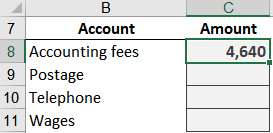Yes … it worked! We fill it down, and bam …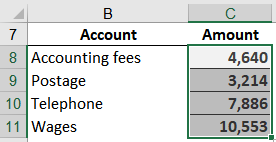Our report is done!

So, that is the general idea of retrieving a related value with VLOOKUP. But before we move on, let’s review a few key points:

• VLOOKUP retrieves a related value
• The 1st argument is the lookup_value, the value we are trying to find
• The 2nd argument is the table_array, which is the lookup table
• The 3rd argument is the col_index_num, which is a whole number that indicates which column within the lookup range has the value you want to return (ie, 2 for the second column within the lookup table, 3 for the third column, etc)
• The 4th argument is range_lookup, and for now, we used FALSE meaning exact match
• It looks vertically (up and down) for a matching value (ie, a vertical lookup, VLOOKUP … you can do a horizontal lookup with HLOOKUP if needed)
• Once it finds its match, it moves to the right to retrieve the related value
• The function looks for the lookup_value in the first (left-most) column within the table_array

So far so good? Great, now let’s examine the 4th argument by looking at the next example.

## Example 2: VLOOKUP TRUE

Let’s say you are not looking for an exact matching value … let’s say you are looking for a value that falls within a range of values. When thinking about text strings, like an account name, this doesn’t make much sense. But, it starts to make more sense when we think about numbers, like values that fall within the range of 100 to 200, or 200 to 300, and so on.

To use a concrete example, let’s say that management has come up with a bonus schedule based on sales. It provides the amount of bonus we pay based on the sales amount. It looks something like this: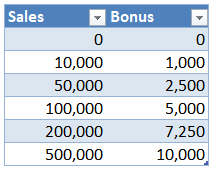This tells us that if the sales amount is between 0 (inclusive) and 10,000 (exclusive), the bonus amount is 0. And if sales fall between 10,000 (inclusive) and 50,000 (exclusive), the bonus amount is 1,000. If sales are between 50,000 (inclusive) and 100,000 (exclusive), the bonus amount is 2,500. And so on.

We want to be able to enter a sales amount into a cell, and have an Excel formula retrieve the corresponding bonus amount. We set up a worksheet accordingly: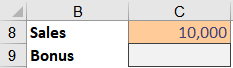To have Excel retrieve the corresponding bonus amount, we can use VLOOKUP. Assuming our bonus table is named Table2, our formula in C9 would look like this:

=VLOOKUP(C8, Table2, 2, TRUE)

Where:

• C8 is the lookup value (the sales amount)
• Table2 is the lookup table (bonus schedule)
• 2 is the column with the value to return (bonus amount)
• TRUE means range lookup (we are not looking for an exact matching sales amount)

We hit Enter and bam, the function returns 1,000 as expected: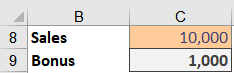We can enter another sales value, and bam, it returns 1,000 as expected:We enter another sales value, and bam … it returns 2,500 as expected:Yay … it works!

So, how does this range lookup logic work exactly? Here’s how I think about it. VLOOKUP starts on the first row in the lookup table and works down, trying to find a row where the sales value (lookup value) is greater than or equal to the current row AND less than the next row.

Here are a few key points about VLOOKUP TRUE:

• The lookup table needs to be sorted in ascending order by the lookup column (first column)
• The 4th argument can be omitted or expressed as TRUE (or a non-zero number) to perform a range lookup
• In practice, I use this mostly with numeric lookup values, but, technically it also works with text strings
• Think of this argument as enabling a range lookup, not as performing an approximate (or fuzzy) match. If you need to do a fuzzy/approximate match instead, check out my partial match or fuzzy match posts.

## Conclusion

Alright my friend, that is a basic introduction to the champion VLOOKUP. In the next post in the series, we’ll meet the challenger SUMIFS. In the meantime, if you’d like to learn more about VLOOKUP, I’ve written another series you can reference here.

Sample file: IntroducingVLOOKUP.xlsx

Get a quick email notice when a new Excel article is available

• This field is for validation purposes and should be left unchanged.1.Eve Peterson
1.Jeff Lenning Post author